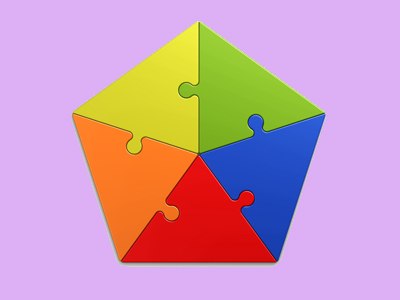A pentagon is not a quadrilateral.

# Shapes (Year 5)

In KS2 Maths, as you move to Year Five, you'll learn more about shapes. You'll know names of 2D shapes, from circles to decagons, and some 3D shapes too. Spotting equilateral, right angle, and isosceles triangles is a breeze now. Get ready for scalene, obtuse triangles, and congruent shapes!

A circle and a triangle are both shapes. Did you know drawing a perfect circle makes you a genius? Try it! Triangles come in types like isosceles, scalene, or equilateral, depending on their angles.

Do you know the difference between an oblong and a rectangle? Can you tell isosceles from a scalene triangle? Test your shape knowledge with this fun quiz!

1.
What is a triangle with no equal sides and no equal angles called?
Isosceles
Equilateral
Scalene
Right angled
Scalene comes from the Greek word for "unequal"
2.
What is an oblong?
A rectangle that is not a square
A rectangle that is a square
A quadrilateral that is not a square
A quadrilateral that is a square
A rectangle is a quadrilateral where all four sides are right angles
3.
What is the name given to a triangle with an angle larger than 90o?
Isosceles
Equilateral
Scalene
Obtuse
Do you remember that angles between 90o and 180o are called obtuse angles?
4.
Which of these shapes is not a quadrilateral?
Oblong
Rectangle
Pentagon
Square
Pentagons have 5 sides - all the other shapes have just 4
5.
What is the name given to the distance from one edge of a circle to the other through the centre?
Circumference
Tangent
Diameter
The diameter is twice the radius
6.
What is the shape of the faces of a regular octahedron?
Square
Octagon
Hexagon
Triangle
A regular octahedron would have equilateral triangles as each of its 8 faces
7.
What is the name given to polygons with all the sides and angles equal?
Irregular polygons
Regular polygons
Isosceles polygons
Equilateral polygons
Irregular polygons are where the sides are not equal
8.
How do we know if two triangles are congruent?
If they both have the same three sides
If they both have a right angle
If they both have the same size angles
If they both have two sides the same
If one shape can become identical to another by using turns, flips or slides but without changing the size, then the two shapes are congruent
9.
If you cut a triangular prism in half, what shape would the new face be?
Circle
Triangle
Square
Hexagon
If you cut a triangle into two with a straight line, at least one of the two pieces will be a triange
10.
What is an octahedron?
3D shape with octagon faces
3D shape with 8 faces
2D shape with 8 sides
2D shape with 8 angles
Remember that 'octa' means 8 and that 'hedron' means a 3D shape
You can find more about this topic by visiting BBC Bitesize - Recognise and describe 3D shapes

Author:  Amanda Swift